# Convective Heat Transfer: One Dimensional (Part - 4) Chemical Engineering Notes | EduRev

## Chemical Engineering : Convective Heat Transfer: One Dimensional (Part - 4) Chemical Engineering Notes | EduRev

The document Convective Heat Transfer: One Dimensional (Part - 4) Chemical Engineering Notes | EduRev is a part of the Chemical Engineering Course Heat Transfer for Engg..
All you need of Chemical Engineering at this link: Chemical Engineering

3.3 Enhanced heat transfer: concept of fins
we have seen that the heat transfer from one fluid to another fluid needs a solid boundary. The rate of heat transfer depends on many factors including the individual heat transfer coefficients of the fluids. The higher the heat transfer coefficients the higher will be the rate of heat transfer. There are many situations where the fluid does not have a high heat transfer coefficient. For example, the heat lost by conduction through a furnace wall must be dissipated to the surrounding by convection through air. The air (or the gas phase in general) has very low heat transfer coefficient, since the thermal conductivities of gases are very low, as compared to the liquid phase. Thus if we make heat transfer device for gas and a liquid (of course separated by a heat conducting wall), the gas side film will offer most of the thermal resistance as compared to the liquid side film. Therefore, to make the heat transfer most effective we need to expose higher area of the conductive wall to the gas side. This can be done by making or attaching fins to the wall of the surface. A fin (in general) is a rectangular metal strip or annular rings to the surface of heat transfer. Thus, a fin is a surface that extends from an object to increase the rate of heat transfer to or from the environment by increasing convections. Fins are sometimes known as extended surface. Figure 3.7 shows photographs of an electric motor with the fins on the motor body and a computer processor with the fins to dissipate the generated heat into the environment. Figure 3.8 shows the different types of finned surfaces.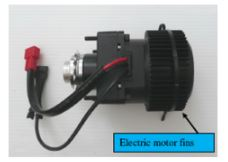(a)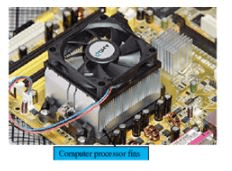(b)

Fig. 3.7. Cooling fins of (a) electric motor, (b) computer processor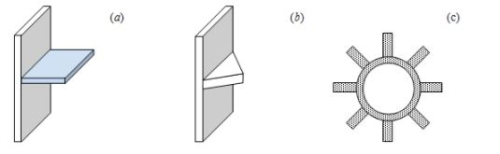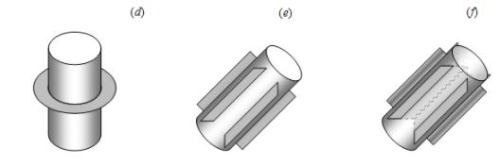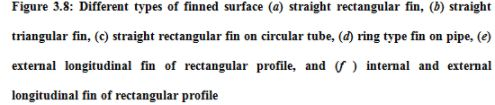Figure 3.9 shows a simple straight rectangular fin on plane wall. The fin is protruded a distance lfrom the wall. The temperature of the plane wall (in fact the base of the fin) is Tw and that of the ambient is T. The distances of the fin are: length l; thickness t; and the breadth b. The heat is conducted through the body by conduction and dissipates to the surrounding by convection. The heat dissipation to the surrounding occurs from both top, bottom, and side surfaces of the fin. Here, it is assumed that the thickness of the fin is small and thus the temperature does not vary in the Y-direction. However, the fin temperature varies in the X-direction only.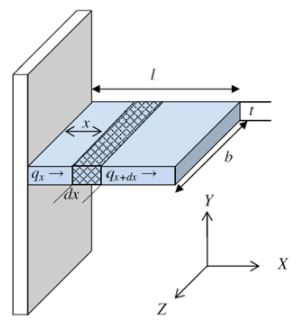Fig. 3.9. 1-D heat conduction and convection through a rectangular fin

Consider a thin element of thickness dx of the fin at a distance x from the fin base. The energy balance on the fin element at steady state is discussed below.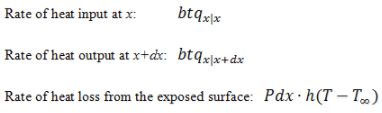where, P is the perimeter [2(b+t)] of the element, T is the local temperature of the fin, h is the film heat transfer coefficient, and bt is the fin area (A) perpendicular to the direction of heat transfer.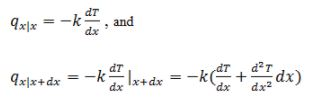Rate of heat input – Rate of heat output – Rate of heat loss = 0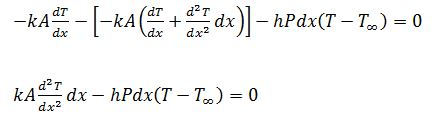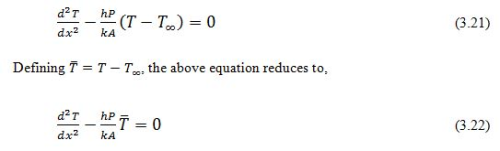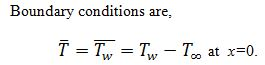However, the other boundary conditions depend on the physical situation of the problem. A few of the typical cases are,
Case I: The fin is very long and thus the temperature at the end of the fin is same as that of the ambient fluid.
Case II: The fin is of finite length and looses heat from its end by convection.

Case III: The end of the fin is insulated so that  at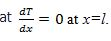Offer running on EduRev: Apply code STAYHOME200 to get INR 200 off on our premium plan EduRev Infinity!

## Heat Transfer for Engg.

46 videos|60 docs|59 tests

,

,

,

,

,

,

,

,

,

,

,

,

,

,

,

,

,

,

,

,

,

;